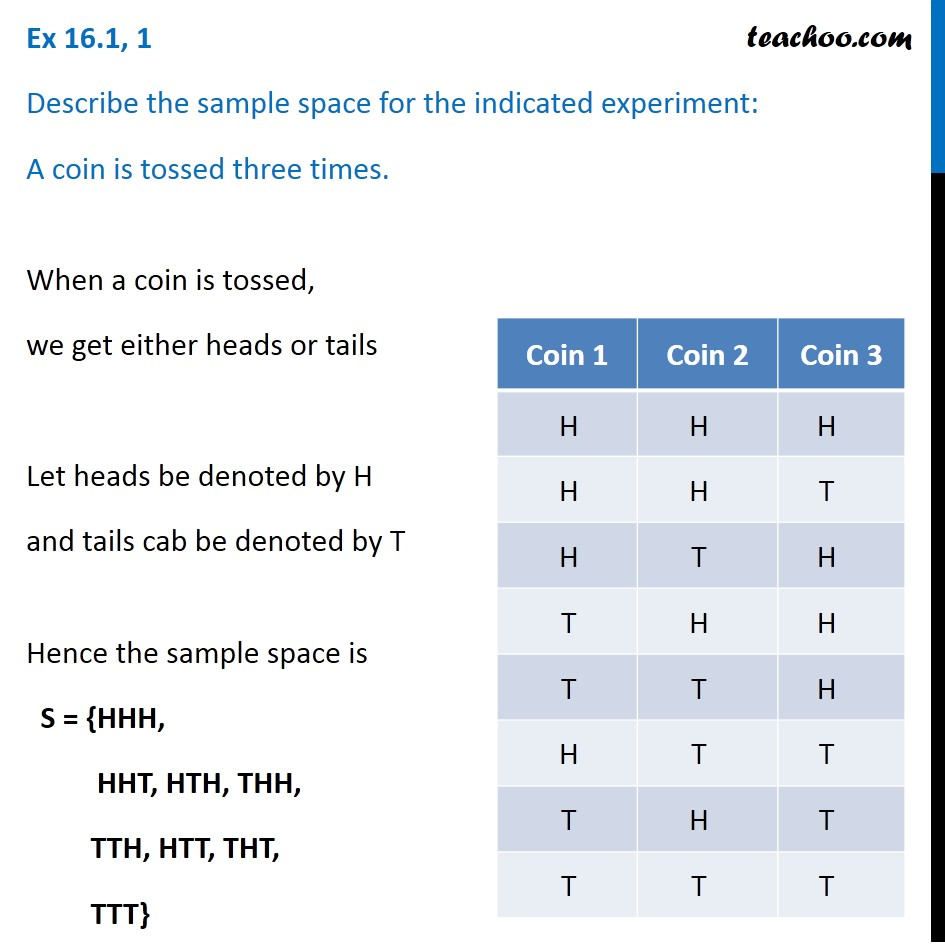1. Chapter 16 Class 11 Probability
2. Concept wise
3. Sample Space

Transcript

Ex 16.1, 1 Describe the sample space for the indicated experiment: A coin is tossed three times. When a coin is tossed, we get either heads or tails Let heads be denoted by H and tails cab be denoted by T Hence the sample space is S = {HHH, HHT, HTH, THH, TTH, HTT, THT, TTT}

Sample Space